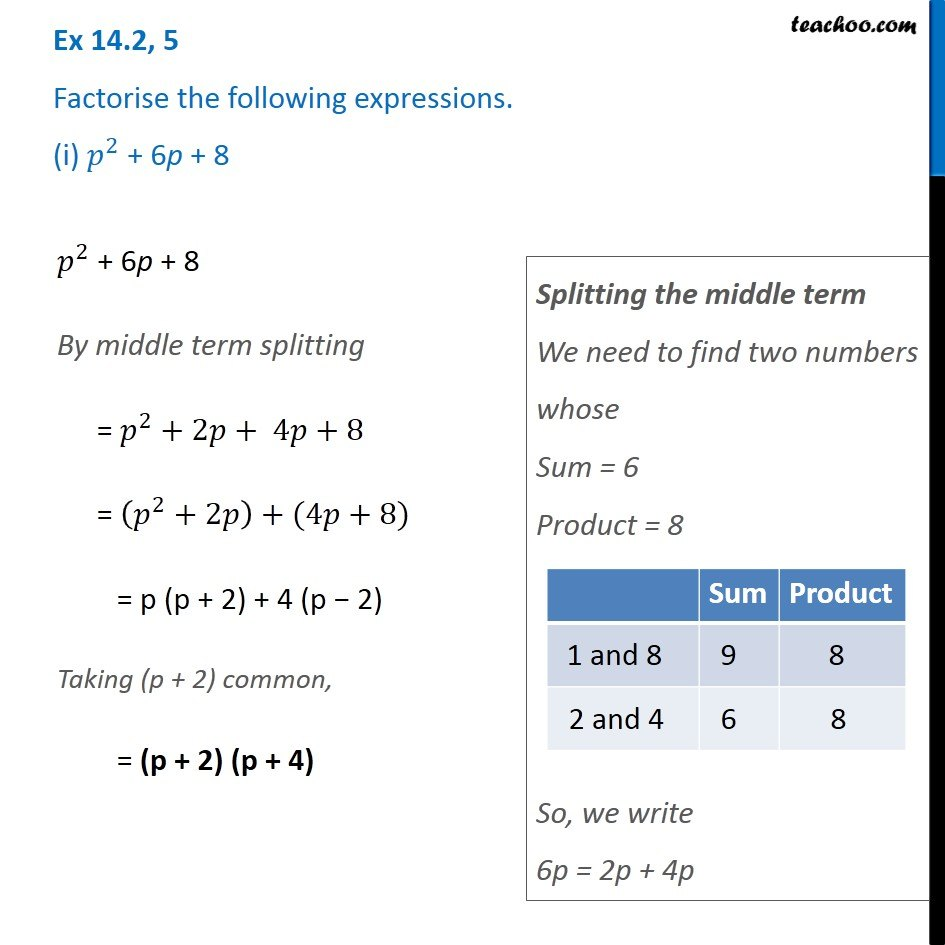Factorisation using splitting middle term

Chapter 14 Class 8 Factorisation
Concept wiseIntroducing your new favourite teacher - Teachoo Black, at only ₹83 per month

### Transcript

Ex 14.2, 5 Factorise the following expressions. (i) 𝑝^2 + 6p + 8 𝑝^2 + 6p + 8 By middle term splitting = 𝑝^2+2𝑝+ 4𝑝+8 = (𝑝^2+2𝑝)+(4𝑝+8) = p (p + 2) + 4 (p − 2) Taking (p + 2) common, = (p + 2) (p + 4) Splitting the middle term We need to find two numbers whose Sum = 6 Product = 8 So, we write 6p = 2p + 4p